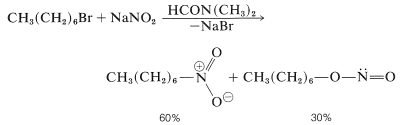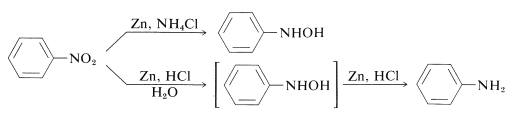# 24.6: Nitro Compounds

$$\newcommand{\vecs}{\overset { \rightharpoonup} {\mathbf{#1}} }$$ $$\newcommand{\vecd}{\overset{-\!-\!\rightharpoonup}{\vphantom{a}\smash {#1}}}$$$$\newcommand{\id}{\mathrm{id}}$$ $$\newcommand{\Span}{\mathrm{span}}$$ $$\newcommand{\kernel}{\mathrm{null}\,}$$ $$\newcommand{\range}{\mathrm{range}\,}$$ $$\newcommand{\RealPart}{\mathrm{Re}}$$ $$\newcommand{\ImaginaryPart}{\mathrm{Im}}$$ $$\newcommand{\Argument}{\mathrm{Arg}}$$ $$\newcommand{\norm}{\| #1 \|}$$ $$\newcommand{\inner}{\langle #1, #2 \rangle}$$ $$\newcommand{\Span}{\mathrm{span}}$$ $$\newcommand{\id}{\mathrm{id}}$$ $$\newcommand{\Span}{\mathrm{span}}$$ $$\newcommand{\kernel}{\mathrm{null}\,}$$ $$\newcommand{\range}{\mathrm{range}\,}$$ $$\newcommand{\RealPart}{\mathrm{Re}}$$ $$\newcommand{\ImaginaryPart}{\mathrm{Im}}$$ $$\newcommand{\Argument}{\mathrm{Arg}}$$ $$\newcommand{\norm}{\| #1 \|}$$ $$\newcommand{\inner}{\langle #1, #2 \rangle}$$ $$\newcommand{\Span}{\mathrm{span}}$$$$\newcommand{\AA}{\unicode[.8,0]{x212B}}$$

## Physical and Spectroscopic Properties

Nitro compounds are a very important class of nitrogen derivatives. The nitro group, $$\ce{-NO_2}$$, like the carboxylate anion, is a hybrid of two equivalent resonance structures:The hybrid structure has a full positive charge on nitrogen and a half-negative charge on each oxygen. This is in accord with the high dipole moments of nitro compounds, which fall between $$3.5 \: \text{D}$$ and $$4.0 \: \text{D}$$, depending upon the nature of $$\ce{R}$$. The polar character of the nitro group results in lower volatility of nitro compounds than ketones of about the same molecular weight; thus the boiling point of nitromethane (MW 61) is $$101^\text{o}$$, whereas 2-propanone (MW 58) has a boiling point of $$56^\text{o}$$. Surprisingly, the water solubility is low; a saturated solution of nitromethane in water is less than $$10\%$$ by weight, whereas 2-propanone is completely miscible with water.

Nitro groups of nitroalkanes can be identified by strong infrared bands at about $$1550 \: \text{cm}^{-1}$$ and $$1375 \: \text{cm}^{-1}$$, whereas the corresponding bands in the spectra of aromatic nitro compounds occur at slightly lower frequencies. A weak $$n \rightarrow \pi^*$$ transition occurs in the electronic spectra of nitroalkanes at around $$270 \: \text{nm}$$; aromatic nitro compounds, such as nitrobenzene, have extended conjugation and absorb at longer wavelengths $$\left( \sim 330 \: \text{nm} \right)$$.

## Preparation of Nitro Compounds

Nitro compounds can be prepared in a number of ways, including the direct substitution of hydrocarbons with nitric acid,

$\ce{RH} + \ce{HONO_2} \rightarrow \ce{RNO_2} + \ce{H_2O}$

by displacement reactions with nitrite ions,

$\ce{RX} + \ce{NO_2^-} \rightarrow \ce{RNO_2} + \ce{X^-}$

and by oxidation of primary amines,

$\ce{RNH_2} \overset{\left[ \ce{O} \right]}{\longrightarrow} \ce{RNO_2}$

Nitration of alkanes is successful only when conducted at high temperatures in the vapor phase. Mixtures of products are invariably obtained (Section 4-6):In contrast, direct nitration of aromatic compounds such as benzene takes place readily in the liquid phase, as discussed in Section 22-4C.

Like other electrophilic substitutions, nitration of a substituted benzene, where the substituent is electron withdrawing ($$\ce{NO_2}$$, $$\ce{CO_2H}$$, $$\ce{CN}$$, and so on; Table 22-6), generally produces the 1,3-isomer. To prepare the 1,4-isomer, less direct routes are necessary - the usual stratagem being to use benzene derivatives with substituent groups that produce the desired orientation on nitration and then to make the necessary modifications in these groups to produce the final product. Thus 1,4-dinitrobenzene cannot be obtained by nitration of nitrobenzene but can be prepared from benzenamine by the sequence shown in Figure 24-5. Benzenamine is converted to $$\ce{N}$$-phenylethanamide (acetanilide) which on nitration yields the 1,4-isomer. Hydrolysis of the amide to 4-nitrobenzenamine and replacement of amino by nitro, using nitrite ion in the presence of cuprous salts, gives 1,4-dinitrobenzene (Section 23-10B). Alternatively, the amino group of 4-nitrobenzenamine can be oxidized to a nitro group by trifluoroperoxyacetic acid. In these syntheses, $$\ce{N}$$-phenylethanamide is nitrated in preference to benzenamine itself because, not only is benzenamine easily oxidized by nitric acid, but the nitration reaction leads to extensive 3-substitution as the result of formation of phenylammonium ion. Another route to 4-nitrobenzenamine is to nitrate chlorobenzene and subsequently replace the chlorine by reaction with ammonia. The nitrations mentioned give mixtures of 2- and 4-isomers, but these usually are easy to separate by distillation or crystallization. The same approach can be used to synthesize 4-nitrobenzoic acid. The methyl group of methylbenzene directs nitration preferentially to the 4 position, and subsequent oxidation with chromic acid yields 4-nitrobenzoic acid:Figure 24-5: Synthetic routes to 1,4-dinitrobenzene.

In some cases it may be necessary to have an activating group to facilitate substitution, which otherwise would be very difficult. The preparation of 1,3,5-trinitrobenzene provides a good example; direct substitution of 1,3-dinitrobenzene requires long heating with nitric acid in fuming sulfuric acid. However, methylbenzene is converted more readily to the trinitro derivative and this substance, on oxidation and decarboxylation (Section 18-4), yields 1,3,5-trinitrobenzene:Acylamino groups also are useful activating groups and have the advantage that the amino groups obtained after hydrolysis of the acyl function can be removed from an aromatic ring by reduction of the corresponding diazonium salt with hypophosphorous acid, preferably in the presence of copper (I) ions. An example is the preparation of 1-methyl-3-nitrobenzene from $$\ce{N}$$-(4-methyl-phenyl)ethanamide (aceto-para-toluidide):Routes to aliphatic nitro compounds include the reaction of an alkyl halide (of good $$S_\text{N}2$$ reactivity) with nitrite ion. Suitable solvents are methylsulfinylmethane [dimethyl sulfoxide, $$\ce{(CH_3)_2SO}$$] and dimethyl methanamide (dimethylformamide). As will be seen from Equation 24-6, formation of the nitrite ester by $$\ce{O}$$- instead of $$\ce{N}$$-alkylation is a competing reaction:Silver nitrite sometimes is used in preference to sodium nitrite, usually in diethyl ether as solvent:Nitromethane can be prepared conveniently by the reaction

$\ce{ClCH_2CO_2H} + \ce{NaNO_2} \underset{-\ce{NaCl}}{\overset{\text{heat}}{\longrightarrow}} \left[ \ce{O_2NCH_2CO_2H} \right] \underset{\text{heat}}{\overset{-\ce{CO_2}}{\longrightarrow}} \ce{O_2NCH_3}$

Displacement reactions with nitrite ion do not work well with aryl halides. However, displacement of the diazonium group is a practical route to nitroarenes (the Sandmeyer reaction), as described in Section 23-10B:

$\ce{ArNH_2} \overset{\ce{HONO}}{\longrightarrow} \ce{Ar} \overset{\oplus}{\ce{N_2}} \overset{\ce{CuNO_2}}{\longrightarrow} \ce{ArNO_2}$

## Reactions of Nitro Compounds

Nitro compounds are quite unstable in the thermodynamic sense; for example, the heat of decomposition of nitromethane, according to the following stoichiometry, is $$67.4 \: \text{kcal mol}^{-1}$$.

$\ce{CH_3NO_2} \rightarrow \frac{1}{2} \ce{N_2} + \ce{CO_2} + \frac{3}{2} \ce{H_2} \: \: \: \: \: \Delta H^0 = -67.4 \: \text{kcal mol}^{-1}$

Advantage is taken of the considerable energies and rapid rates of reactions such as this in the commercial use of nitro compounds as explosives. With some nitro compounds, such as TNT, there is a further advantage of low shock sensitivity.TNT is not detonated easily by simple impact and even burns without exploding. However, once detonation starts, decomposition is propagated rapidly. The characteristics of reasonable handling stability and high thermodynamic potential make nitro compounds particularly useful. Other polynitro compounds that are useful as explosives include PETN (Section 17-3C), cyclonite (Section 16-4C), picric acid, and tetryl:An important characteristic of aromatic polynitro compounds is their ability to form "charge-transfer" complexes with aromatic hydrocarbons, especially those that are substituted with alkyl groups. Complexes of 2,4,6-trinitrobenzenol (picric acid) and aromatic hydrocarbons often are crystalline solids, which are useful for the separation, purification, and identification of aromatic hydrocarbons. These substances are called "hydrocarbon picrates", but the name is misleading because they are not actually salts. Furthermore, similar complexes are formed between aromatic hydrocarbons and trinitrobenzene, which demonstrates that the nitro groups rather than the hydroxyl group are essential to complex formation. The binding in these complexes resembles that in the $$\pi$$ complexes of halogens with alkenes and benzene (Sections 22-4D and 10-3C) and results from attractive forces between electron-rich and electron-poor substances. The descriptive name - charge-transfer complex - suggests that the complex has VB structures involving transfer of an electron from the donor (electron-rich) molecule to the acceptor (electron-poor) molecule. The name $$\pi$$ complex also is used because, usually at least, one component of the complex has a $$\pi$$-electron system. Charge-transfer or $$\pi$$ complexes between polynitro compounds and aromatic hydrocarbons appear to give sandwich-type structures with the aromatic rings in parallel planes, although not necessarily centered exactly over one another:Charge-transfer complexes are almost always more highly colored than their individual components. A spectacular example is benzene and tetracyanoethene, each of which separately is colorless, but which give a bright-orange complex when mixed. A shift toward longer wavelengths of absorption, relative to their components, is to be expected for charge-transfer complexes because of the enhanced possibility for stabilization of the excited state through electron delocalization involving both components.

Reduction of nitro compounds occurs readily with a variety of reducing agents and such reductions afford a particularly useful synthesis of aromatic amines (Section 23-12B):The reduction of a nitro compound to an amine requires six equivalents of reducing agent:

$\ce{R-NO_2} + 6 \ce{H}^\oplus + 6 \ce{e}^\ominus \rightarrow \ce{RNH_2} + 2 \ce{H_2O}$

One would not expect such a reduction to occur in a single step. Indeed, reduction is stepwise and proceeds through a string of intermediates, which, with strong reducing agents in acid solution, have at most a transient existence. The intermediates formed successively from $$\ce{RNO_2}$$ by increments of two equivalents of reducing agent are nitroso compounds, $$\ce{R-N=O}$$, and $$\ce{N}$$-substituted azanols (hydroxylamines), $$\ce{RNHOH}$$:

$\ce{RNO_2} \underset{-\ce{H_2O}}{\overset{2 \left[ \ce{H} \right]}{\longrightarrow}} \ce{RN=O} \overset{2 \left[ \ce{H} \right]}{\longrightarrow} \ce{RNHOH} \underset{-\ce{H_2O}}{\overset{2 \left[ \ce{H} \right]}{\longrightarrow}} \ce{RNH_2}$

Thus $$\ce{N}$$-aryl-substituted azanols can be obtained directly from the corresponding nitro compounds with zinc and ammonium chloride solution. However, zinc and hydrochloric acid gives the amine:The difference between these reactions is in the reduction rates associated with the acidity of the solution. Ammonium chloride is a much weaker acid than $$\ce{HCl}$$; the pH of ammonium chloride solutions is around 6.

Oxidation of the $$\ce{N}$$-arylazanols under controlled conditions yields nitroso compounds. This reaction is not unlike the oxidation of alcohols to ketones (Section 15-6B):Reduction of aryl nitro compounds with less-powerful reducing agents, especially in alkaline media, gives what may appear to be a mysterious conglomerate of bimolecular reduction products. For example, with nitrobenzene,All of these substances can be reduced to benzenamine with tin and hydrochloric acid. As a result, each could be, but not necessarily is, an intermediate in the reduction of nitro compounds to amines. Formation of the bimolecular reduction products is the result of base-induced reactions between nitroso compounds and azanols or amines and possibly further reduction of the initially produced substances.

Several polynitrobenzene derivatives have important herbicidal uses. Examples are $$\ce{N}^3$$, $$\ce{N}^3$$-diethyl-6-trifluoromethyl-2,4-dinitro-1,3-benzenediamine and $$\ce{N}$$,$$\ce{N}$$-dipropyl-4-trifluoromethyl-2,6-dinitrobenzenamine:These substances when mixed with soil kill weed seedlings but not crop plants such as cotton, soybeans, and peanuts. The activity is high; normally only about $$0.08 \: \text{g m}^{-2}$$ is required for good weed control.

The most important reactions of nitroalkanes are those involving the $$\alpha$$ hydrogens of the primary and secondary compounds. For example, nitromethane is sufficiently acidic to dissolve in aqueous hydroxide solutions. The anion so produced has an electronic structure analogous to the nitrate anion:An interesting property of this ion is that when solutions of it are acidified, an unstable, rather strongly acidic isomer of nitromethane (called the aci form) is produced, which slowly reverts to the more stable nitro form:Similar changes take place in the acidification of the enol salt of a carbonyl compound, the principal difference being the much longer life of the aci-nitro compound compared to that of an enol of a simple ketone (see Section 17-1B).

Primary and secondary nitro compounds undergo aldol additions and Michael additions with suitable carbonyl compounds and basic catalysts:Unfortunately, alkylation reactions analogous to the base-catalyzed alkylation of carbonyl compounds generally are not useful for the synthesis of higher nitro compounds, because $$\ce{C}$$-alkylation of the conjugate bases of primary nitro compounds is slower than $$\ce{O}$$-alkylation.

## Contributors and Attributions

John D. Robert and Marjorie C. Caserio (1977) Basic Principles of Organic Chemistry, second edition. W. A. Benjamin, Inc. , Menlo Park, CA. ISBN 0-8053-8329-8. This content is copyrighted under the following conditions, "You are granted permission for individual, educational, research and non-commercial reproduction, distribution, display and performance of this work in any format."

This page titled 24.6: Nitro Compounds is shared under a not declared license and was authored, remixed, and/or curated by John D. Roberts and Marjorie C. Caserio.